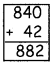Tamilnadu State Board New Syllabus Samacheer Kalvi 4th Maths Guide Pdf Term 3 Chapter 2 Numbers Ex 2.7 Textbook Questions and Answers, Notes.

## Tamilnadu Samacheer Kalvi 4th Maths Solutions Term 3 Chapter 2 Numbers Ex 2.7

Simplify the following multiplication facts by adding partial products.
Question 1.
9 × 42
42 = 40 + 2
40 × 9 = 360
2 × 9 = 18
9 × 42 = 360 + 18 = 378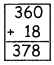Question 2.
3 × 78
78 = 70 + 8
70 × 3 = 210
8 × 3 = 24
3 × 78 = 210 + 24 = 234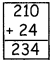Question 3.
36 × 12
36 = 30 + 6
30 × 12 = 360
6 × 12 = 72
36 × 12 = 360 + 72 = 432Question 4.
18 × 19
19 = 10 + 9
10 × 18 = 180
18 × 9 = 162
180 + 162 = 342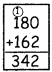Question 5.
68 × 31
68 = 60 + 8
60 × 31 = 1860
8 × 31 = 248
68 × 31 = 1860 + 248 = 2108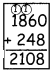Question 6.
42 × 21